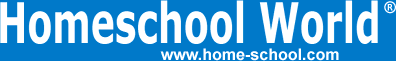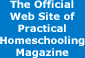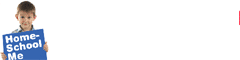Home Curriculum Catalog Articles Contests Events Groups Forum News ContactGetting Started Preschool/K Elementary Middle School High School College & Career Prep Special Needs Group Activities Research FAQs# Graph This

By Bill Pride
Printed in Practical Homeschooling #75, 2007.Graphing calculators can do all kinds of incredibly fascinating things.In my last article I explored the idea of using calculators for teaching elementary-school mathematics and concluded that calculators are not useful for teaching arithmetic.

What about higher mathematics, such as algebra, trigonometry, and calculus? More and more high-school math courses, even math programs widely used by homeschoolers, include or require the use of graphing calculators.

Why is this? Certainly, there must be a good reason why all these curriculum writers think it is worthwhile to include the use of a graphing calculator in their courses.

From the teacher’s point of view, there is a certain amount of “technoglam” associated with having a high-tech curriculum. From the calculator manufacturer’s point of view, including calculators in every major math curriculum is a very financially desirable goal. Texas Instruments sold its 40 millionth graphing calculator in 2006. At \$150–\$200 apiece, that comes to multiple billions, and that doesn’t include the billions earned by Casio, Hewlett-Packard, and the other manufacturers.

## Can You Learn Higher Math Without a Graphing Calculator?

This question really doesn’t need to be asked. Most of the higher math taught is colleges was formulated long before the invention of the pocket calculator. Most mathematics doesn’t require a calculator and many mathematicians detest having to use either a calculator or a computer for anything.

To a mathematician, finding a proven method to get the answer and an exact mathematical expression for the answer is enough—you don’t need to compute the decimal value of the answer to 10 decimal places.

For example, the equation:

x2 + 2x - 2 = 0

has two answers that you can find by mathematically solving the equation:

-1 + √3 and -1 - √3. Tracing the graph using a graphing calculator you get an answer around .732 for one of these and around -2.732 for the other. With judicious playing around with the calculator’s scale and trace functions, you can get answers to higher precision than that. You will have found the correct numerical solutions to the equation, but that’s not what math is all about.

Math is learning how to solve problems and come up with an exact expression for the answer. You only compute the decimal approximation of the answer when the problem requires it.

A number of articles quote the fact that there is a positive correlation between the number of days per week spent using a graphing calculator and test scores. This is misleading. Correlations may sound significant, but that can be deceptive.

First, in this case, the correlation only holds for some countries. Other countries had the exact opposite result. Also, a corellation between two things does not mean that one thing causes another. A positive correlation only means that the two quantities go up together and go down together. For example, in St. Louis city, there is a correlation between the amount of ice cream consumed and the number of murders committed. Do you think eating ice cream causes murder, or the other way around? No, both are results of the summer heat.

Use of a graphing calculator does not necessarily cause better test scores. Maybe the greater number of days using a graphing calculator only means a greater number of days studying mathematics and that is the cause. Or maybe students who do well in math just like to play with their calculators and the cause of the good scores is something else entirely. Don’t be fooled by correlations.

You don’t need a calculator to learn mathematics. For example, to learn order of operations in Algebra 1, you learn the mnemonic “PEMDAS” (parentheses, exponents, multiplication and division, addition and subtraction). You need to know PEMDAS in order to accurately use a graphing calculator. The calculator doesn’t help you learn it.

When I am doing problems in statistic, often the writeup for a problem will take multiple sheets of paper. The part where I use my calculator is the last line where I actually need to run the numbers. The calculator did not help me until I reached that point and a scientific calculator costing less than \$20 can take care of the last line.

Graphing calculators actually can hinder math learning in a number of ways:

• The calculator doesn’t help you learn to draw and understand graphs. People who learn by doing—“kinesthetic learners”—do not benefit from letting a graphing calculator do it for them.
• Graphs on a graphing calculator have no permanence. If you want to go back and study what you did a week later, a hard copy graph is a necessity.
• You have no way to check your answer on a calculator if you don’t know approximately what answer to expect. If you were inept at entering a calculation, you will never know. The old computer expression G.I.G.O. (garbage in, garbage out) applies even more to calculators.
• Calculators lead to passivity and lack of creativity. Mathematicians should always be thinking, “Can I prove the validity of this result?” Using calculators, you tend to accept whatever answer the calculator comes up with.
• Use of graphing calculators is causing curriculum to be designed around the calculators. Time spent learning how to use a graphing calculator is time not spent learning how to solve math problems the traditional way. So far, this has already caused high-school calculus texts to be redesigned, and similar changes have been proposed for algebra and advanced math.

## What is a Graphing Calculator Good For?

As a Math Manipulative. Just like you can use Cuisenaire Rods to help you conceptualize how to add and subtract, the graphing calculator can help you discover the shape of various graphs and how the graphs change when you change the equation. You can illustrate the idea of roots, continuity, differentials, etc. These concepts can also be illustrated with diagrams in a book. However, in my experience, I only really comprehend the important details of the shape of the graph of a function when I plot the points and connect the dots myself.

For Checking Your Work. A calculator is the fastest way to check the result of a long computation. It lets the student check himself, saving time for a harried homeschool teacher.

As a Computational Tool. I do not enjoy unnecessary work, especially when the product is inferior to what I can get using tools with far less effort. It’s easier to use a rotary saw instead of a hand saw, to use a calculator instead of pencil and paper, and to use a graphing calculator with a multi-line display instead of a scientific calculator. On the other hand, it may be worth it to put up with a little inconvenience to save the \$130 difference in price.

Note also, the more advanced graphing calculators are too powerful. The data storage capabilities of programmable calculators make it possible for them to be used to cheat on exams, so many schools forbid their use in tests. I had to buy a less capable scientific calculator for use on my tests in college.

As a Substitute for Math Tables. Back in the stone ages before scientific calculators and graphing calculators, if you wanted to find sin(23°) you would have to look it up in trigonometric tables. I don’t think anyone even publishes these anymore. All the copies on Amazon appear to be from out-of-print editions.

To use the table, you would look up the angle in degrees (and in some editions, minutes, and seconds), in the rightmost column of the table. Each row contains the angle in radians and the value of the sine, cosine and tangent of the angle. To get secant, cosecant, and cotangent, you would have to take the reciprocal of the appropriate function from the table.

It is much easier to type in an angle, push one button, and read the result. But for that you don’t need a graphing calculator. A scientific calculator will do the job at a much lower price.

## Summing Up

Graphing calculators have their place. . . . but I don’t believe they belong in high school math classes. The calculator distracts too much from actually learning the mathematics. Also, learning to use a graphing calculator is not a trivial process. The e-book of instructions for my TI-86 is 419 pages long.

I would urge you to choose math courses that minimize the overuse of graphing calculators. BJU’s Precalculus text is a good example. It specifically tells students not to use calculators for most exercises, and has a separate appendix on graphing calculator use. Then, the summer before entering college, buy a “For Dummies” book or a how-to DVD and learn how to use your graphing calculator’s many features.Terms of Use   Privacy Policy Copyright ©1993-2023 Home Life, Inc.